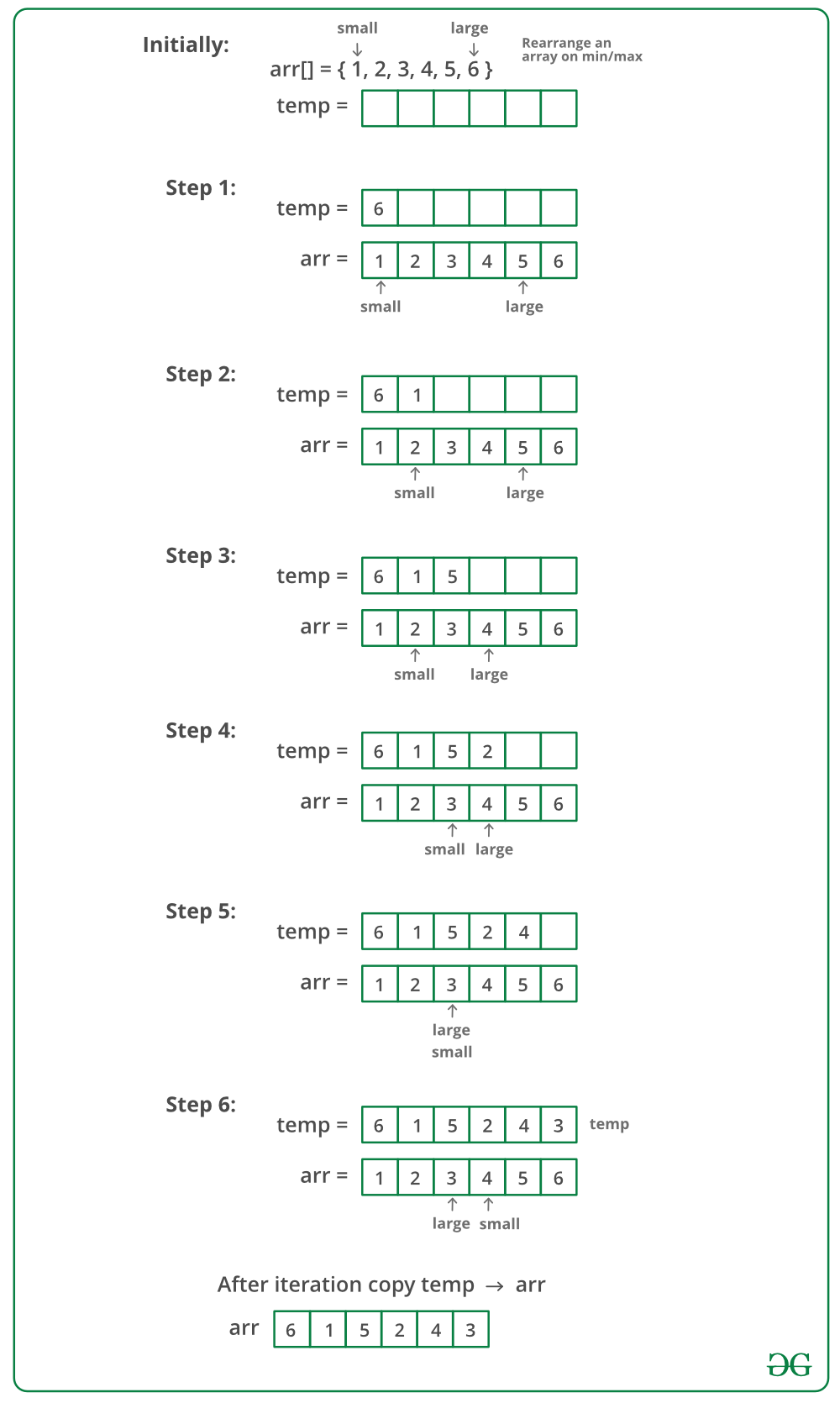GeeksforGeeks App
Open AppBrowser
Continue

# Rearrange an array in maximum minimum form using Two Pointer Technique

Given a sorted array of positive integers, rearrange the array alternately i.e first element should be a maximum value, at second position minimum value, at third position second max, at fourth position second min, and so on.

Examples:

Input: arr[] = {1, 2, 3, 4, 5, 6, 7}
Output: arr[] = {7, 1, 6, 2, 5, 3, 4}

Input: arr[] = {1, 2, 3, 4, 5, 6}
Output: arr[] = {6, 1, 5, 2, 4, 3}

## Rearrange an array in maximum minimum form using Two Pointers:

The idea is to use an auxiliary array. We maintain two pointers one to the leftmost or smallest element and the other to the rightmost or largest element. We move both pointers toward each other and alternatively copy elements at these pointers to an auxiliary array. Finally, we copy the auxiliary array back to the original array.Below is the implementation of the above approach:

## C++

 `// C++ program to rearrange an array in minimum``// maximum form``#include ``using` `namespace` `std;`` ` `// Prints max at first position, min at second position``// second max at third position, second min at fourth``// position and so on.``void` `rearrange(``int` `arr[], ``int` `n)``{``    ``// Auxiliary array to hold modified array``    ``int` `temp[n];`` ` `    ``// Indexes of smallest and largest elements``    ``// from remaining array.``    ``int` `small = 0, large = n - 1;`` ` `    ``// To indicate whether we need to copy remaining``    ``// largest or remaining smallest at next position``    ``int` `flag = ``true``;`` ` `    ``// Store result in temp[]``    ``for` `(``int` `i = 0; i < n; i++) {``        ``if` `(flag)``            ``temp[i] = arr[large--];``        ``else``            ``temp[i] = arr[small++];`` ` `        ``flag = !flag;``    ``}`` ` `    ``// Copy temp[] to arr[]``    ``for` `(``int` `i = 0; i < n; i++)``        ``arr[i] = temp[i];``}`` ` `// Driver code``int` `main()``{``    ``int` `arr[] = { 1, 2, 3, 4, 5, 6 };``    ``int` `n = ``sizeof``(arr) / ``sizeof``(arr);`` ` `    ``cout << ``"Original Array\n"``;``    ``for` `(``int` `i = 0; i < n; i++)``        ``cout << arr[i] << ``" "``;`` ` `    ``rearrange(arr, n);`` ` `    ``cout << ``"\nModified Array\n"``;``    ``for` `(``int` `i = 0; i < n; i++)``        ``cout << arr[i] << ``" "``;``    ``return` `0;``}`

## Java

 `// Java program to rearrange an array in minimum``// maximum form`` ` `import` `java.util.Arrays;`` ` `public` `class` `GFG ``{``    ``// Prints max at first position, min at second position``    ``// second max at third position, second min at fourth``    ``// position and so on.``    ``static` `void` `rearrange(``int``[] arr, ``int` `n)``    ``{``        ``// Auxiliary array to hold modified array``        ``int` `temp[] = arr.clone();`` ` `        ``// Indexes of smallest and largest elements``        ``// from remaining array.``        ``int` `small = ``0``, large = n - ``1``;`` ` `        ``// To indicate whether we need to copy remaining``        ``// largest or remaining smallest at next position``        ``boolean` `flag = ``true``;`` ` `        ``// Store result in temp[]``        ``for` `(``int` `i = ``0``; i < n; i++) {``            ``if` `(flag)``                ``arr[i] = temp[large--];``            ``else``                ``arr[i] = temp[small++];`` ` `            ``flag = !flag;``        ``}``    ``}`` ` `    ``// Driver code``    ``public` `static` `void` `main(String[] args)``    ``{``        ``int` `arr[] = ``new` `int``[] { ``1``, ``2``, ``3``, ``4``, ``5``, ``6` `};`` ` `        ``System.out.println(``"Original Array "``);``        ``System.out.println(Arrays.toString(arr));`` ` `        ``rearrange(arr, arr.length);`` ` `        ``System.out.println(``"Modified Array "``);``        ``System.out.println(Arrays.toString(arr));``    ``}``}`

## Python3

 `# Python program to rearrange an array in minimum``# maximum form`` ` `# Prints max at first position, min at second position``# second max at third position, second min at fourth``# position and so on.`` ` ` ` `def` `rearrange(arr, n):``    ``# Auxiliary array to hold modified array``    ``temp ``=` `n``*``[``None``]`` ` `    ``# Indexes of smallest and largest elements``    ``# from remaining array.``    ``small, large ``=` `0``, n``-``1`` ` `    ``# To indicate whether we need to copy remaining``    ``# largest or remaining smallest at next position``    ``flag ``=` `True`` ` `    ``# Store result in temp[]``    ``for` `i ``in` `range``(n):``        ``if` `flag ``is` `True``:``            ``temp[i] ``=` `arr[large]``            ``large ``-``=` `1``        ``else``:``            ``temp[i] ``=` `arr[small]``            ``small ``+``=` `1`` ` `        ``flag ``=` `bool``(``1``-``flag)`` ` `    ``# Copy temp[] to arr[]``    ``for` `i ``in` `range``(n):``        ``arr[i] ``=` `temp[i]``    ``return` `arr`` ` ` ` `# Driver code``arr ``=` `[``1``, ``2``, ``3``, ``4``, ``5``, ``6``]``n ``=` `len``(arr)``print``(``"Original Array"``)``print``(arr)``print``(``"Modified Array"``)``print``(rearrange(arr, n))`` ` `# This code is contributed by Pratik Chhajer`

## C#

 `// C# program to rearrange``// an array in minimum``// maximum form``using` `System;`` ` `class` `GFG {`` ` `    ``// Prints max at first position,``    ``// min at second position second``    ``// max at third position, second``    ``// min at fourth position and so on.``    ``static` `void` `rearrange(``int``[] arr, ``int` `n)``    ``{``        ``// Auxiliary array to``        ``// hold modified array``        ``int``[] temp = ``new` `int``[n];`` ` `        ``// Indexes of smallest``        ``// and largest elements``        ``// from remaining array.``        ``int` `small = 0, large = n - 1;`` ` `        ``// To indicate whether we``        ``// need to copy remaining``        ``// largest or remaining``        ``// smallest at next position``        ``bool` `flag = ``true``;`` ` `        ``// Store result in temp[]``        ``for` `(``int` `i = 0; i < n; i++) {``            ``if` `(flag)``                ``temp[i] = arr[large--];``            ``else``                ``temp[i] = arr[small++];`` ` `            ``flag = !flag;``        ``}`` ` `        ``// Copy temp[] to arr[]``        ``for` `(``int` `i = 0; i < n; i++)``            ``arr[i] = temp[i];``    ``}`` ` `    ``// Driver Code``    ``static` `void` `Main()``    ``{``        ``int``[] arr = { 1, 2, 3, 4, 5, 6 };`` ` `        ``Console.WriteLine(``"Original Array"``);``        ``for` `(``int` `i = 0; i < arr.Length; i++)``            ``Console.Write(arr[i] + ``" "``);`` ` `        ``rearrange(arr, arr.Length);`` ` `        ``Console.WriteLine(``"\nModified Array"``);``        ``for` `(``int` `i = 0; i < arr.Length; i++)``            ``Console.Write(arr[i] + ``" "``);``    ``}``}`` ` `// This code is contributed``// by Sam007`

## PHP

 ``

## Javascript

 ``

Output

```Original Array
1 2 3 4 5 6
Modified Array
6 1 5 2 4 3 ```

Time Complexity: O(N), Iterating over the array of size N 2 times.
Auxiliary Space: O(N), since N extra space has been taken.

Exercise: How to solve this problem if extra space is not allowed?
Rearrange an array in maximum minimum form | Set 2 (O(1) extra space)

My Personal Notes arrow_drop_up